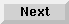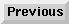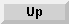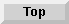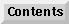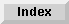7.4 Floating-point numbers

7.4.5 A known problem

Type and representation information is not being propagated from a declared variable ofmultiple-value-setq ormultiple-value-bind onto the expression supplying the value(s). Therefore, code such as the following fails to be optimized:

(defun baz (x y)
(declare (fixnum x y))
(multiple-value-bind (z w)
(round x y)
(declare (fixnum z w))
(list (+ z 1) (+ w 1.0))))

Below are two examples of code that is successfully optimized:

(defun bar (x y) (declare (fixnum x y))
(multiple-value-bind (z w)
(the (values fixnum fixnum) (round x y))
(list (+ z 1) (+ w 1.0))))

(defun foo (x y) (declare (fixnum x y)) (let ((z (round x y))) (declare (fixnum z)) (+ z 1)))

In some cases, it may be just as efficient to re-calculate the remainder, for example, as follows:

(defun foobar  (x y)
(declare (float x y))
(let* ((quo (round x y))
(rem (- x (* y (float quo)))))
(declare (fixnum quo)
(float rem))
(list (+ quo 1) (+ rem 1.0))))

The Advanced User's Guide - 9 SEP 1996Generated with Harlequin WebMaker